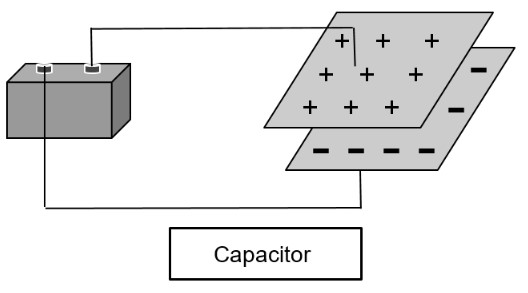# Capacitance Calculator

To find the value of capacitance of a capacitor, enter the values of charge and voltage in the capacitance calculator.

Formula:
C = QV
C
V

Give Us Feedback

This capacitance calculator calculates the capacitance, the ability to store charge. You can use this calculator for the calculation of capacitance for both series and parallel plate capacitors.

## What is Capacitance?

Capacitance can be defined as,

“Capacitance is the ability of a component or circuit to collect and store energy in the form of an electrical charge.”Capacitance is a characteristic of a capacitor, one of the three important parts of a circuit. The effect of capacitance can be used in logic gates, power supplies, RF circuits e.t.c.

Aside from capacitors, there can be levels of capacitance in resistors, inductors, Printed circuits, and other things.

## Capacitance formula

The equation used for capacitance is:

Q = CV

In this equation:

• Q is capacitance
• C is the capacitance
• V is voltage

This formula is rearranged to compute capacitance such as:

C = Q/V

## How to calculate capacitance?

Example

A circuit consists of a battery of 9 volts. The charge stored in its capacitor is 18 coulombs. What is the capacitance of its capacitor?

Solution:

Step 1: Identify the values.

Q = 18 C

V =  9 V

Step 2: Put the values in the capacitance equation given above.

C = Q / V

C = 18 / 9

## How to increase capacitance?

Analyzing the capacitance equation, we can learn how to increase its value.

### Through plate size

Capacitance is directly proportional to the amount of charge. So by increasing plates’ size and in turn increasing the amount of charge, we can increase the capacitance.

### Decreasing voltage

Since capacitance is inversely proportional to voltage, we can increase its value if the voltage is decreased.

### Math Tools